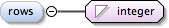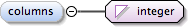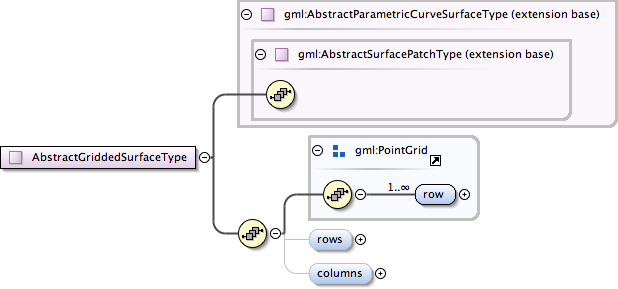### Showing:

 Annotations Diagrams Properties Source Used by
Element gml:AbstractGriddedSurfaceType / gml:rows
Namespace http://www.opengis.net/gml
Annotations
 ```The attribute rows gives the number of rows in the parameter grid.```
DiagramType integer
Properties
 content: simple minOccurs: 0
Source
 ``` The attribute rows gives the number of rows in the parameter grid. ```
Schema location http://schemas.opengis.net/gml/3.1.1/base/geometryPrimitives.xsd
Element gml:AbstractGriddedSurfaceType / gml:columns
Namespace http://www.opengis.net/gml
Annotations
 ```The attribute columns gives the number of columns in the parameter grid.```
DiagramType integer
Properties
 content: simple minOccurs: 0
Source
 ``` The attribute columns gives the number of columns in the parameter grid. ```
Schema location http://schemas.opengis.net/gml/3.1.1/base/geometryPrimitives.xsd
Complex Type gml:AbstractGriddedSurfaceType
Namespace http://www.opengis.net/gml
Annotations
 ```A gridded surface is a parametric curve surface derived from a rectangular grid in the parameter space. The rows from this grid are control points for horizontal surface curves; the columns are control points for vertical surface curves. The working assumption is that for a pair of parametric co-ordinates (s, t) that the horizontal curves for each integer offset are calculated and evaluated at "s". The defines a sequence of control points: cn(s) : s 1 .....columns From this sequence a vertical curve is calculated for "s", and evaluated at "t". In most cases, the order of calculation (horizontal-vertical vs. vertical-horizontal) does not make a difference. Where it does, the horizontal- vertical order shall be the one used. Logically, any pair of curve interpolation types can lead to a subtype of GriddedSurface. The following clauses define some most commonly encountered surfaces that can be represented in this manner.```
DiagramType extension of gml:AbstractParametricCurveSurfaceType
Type hierarchy
Used by
 Element gml:_GriddedSurface Complex Types gml:ConeType, gml:CylinderType, gml:SphereType
Model gml:row+ , gml:rows{0,1} , gml:columns{0,1}
Children gml:columns, gml:row, gml:rows
Source
 ``` A gridded surface is a parametric curve surface derived from a rectangular grid in the parameter space. The rows from this grid are control points for horizontal surface curves; the columns are control points for vertical surface curves. The working assumption is that for a pair of parametric co-ordinates (s, t) that the horizontal curves for each integer offset are calculated and evaluated at "s". The defines a sequence of control points: cn(s) : s 1 .....columns From this sequence a vertical curve is calculated for "s", and evaluated at "t". In most cases, the order of calculation (horizontal-vertical vs. vertical-horizontal) does not make a difference. Where it does, the horizontal- vertical order shall be the one used. Logically, any pair of curve interpolation types can lead to a subtype of GriddedSurface. The following clauses define some most commonly encountered surfaces that can be represented in this manner. This is the double indexed sequence of control points, given in row major form. NOTE! There in no assumption made about the shape of the grid. For example, the positions need not effect a "21/2D" surface, consecutive points may be equal in any or all of the ordinates. Further, the curves in either or both directions may close. The attribute rows gives the number of rows in the parameter grid. The attribute columns gives the number of columns in the parameter grid. ```
Schema location http://schemas.opengis.net/gml/3.1.1/base/geometryPrimitives.xsd